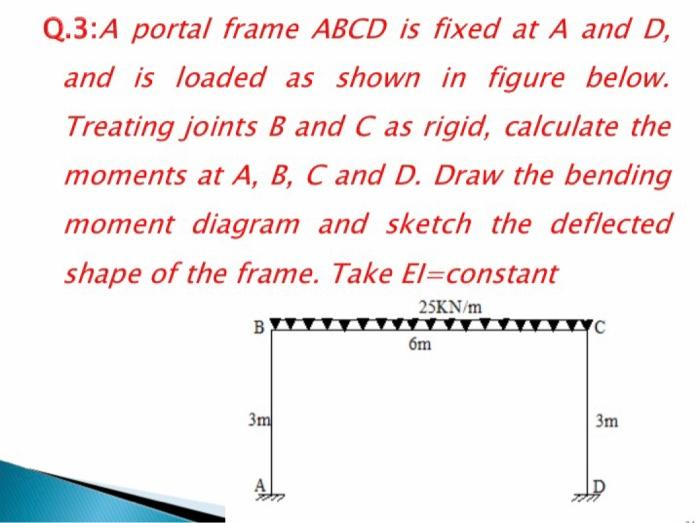# Question Q.3:A portal frame ABCD is fixed at A and D, and is loaded as shown in figure below. Treating joints B and C as rigid, calculate the moments at A, B, C and D. Draw the bending moment diagram and sketch the deflected shape of the frame. Take El=constant 25KN/m бm B 3m 3mRPPPCX The Asker · Civil EngineeringTranscribed Image Text: Q.3:A portal frame ABCD is fixed at A and D, and is loaded as shown in figure below. Treating joints B and C as rigid, calculate the moments at A, B, C and D. Draw the bending moment diagram and sketch the deflected shape of the frame. Take El=constant 25KN/m бm B 3m 3m
More
Transcribed Image Text: Q.3:A portal frame ABCD is fixed at A and D, and is loaded as shown in figure below. Treating joints B and C as rigid, calculate the moments at A, B, C and D. Draw the bending moment diagram and sketch the deflected shape of the frame. Take El=constant 25KN/m бm B 3m 3m